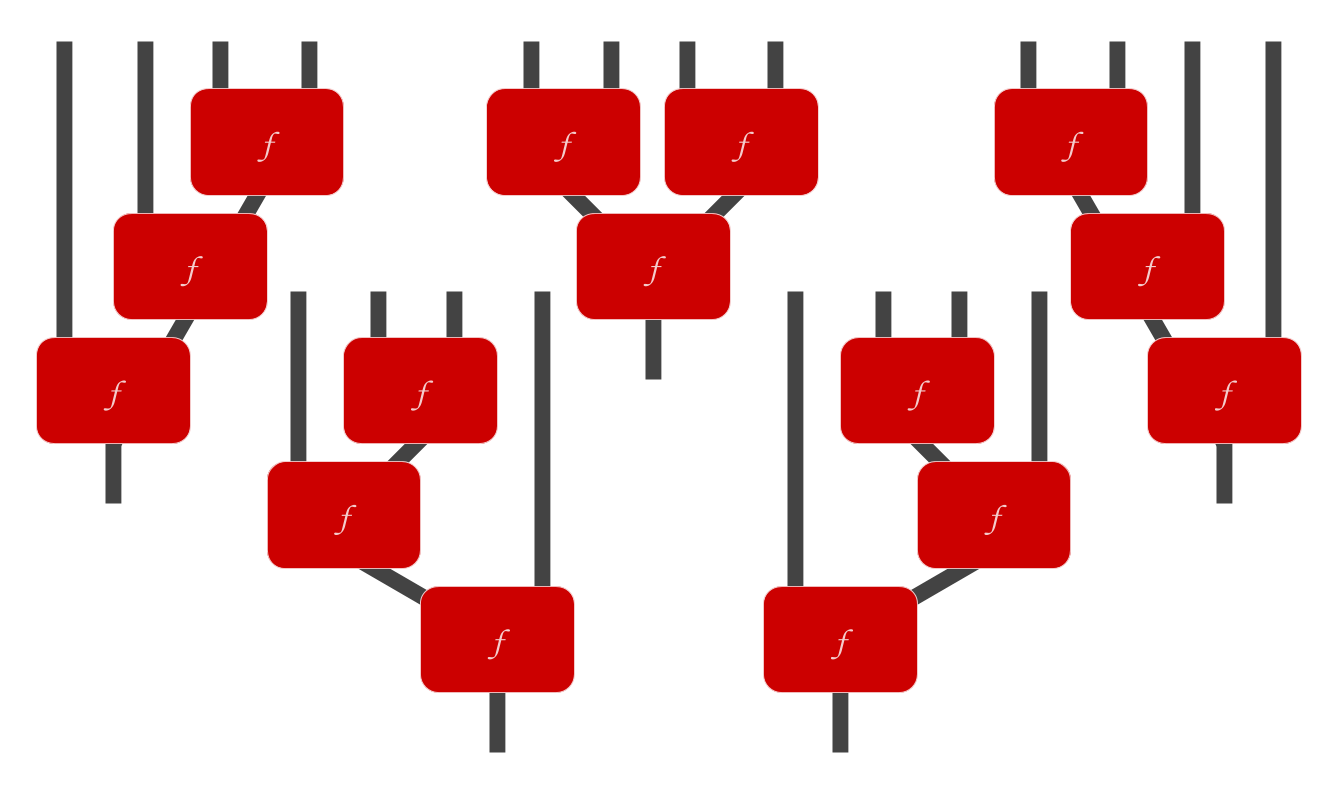# Generalized associative law

Given an associative operator $$\cdot$$ and a list $$[a, b, c, \ldots]$$ of parameters, all ways of reducing the list to a single element by combining adjacent pairs yield the same result. In other words, while associativity only talks about the behavior of $$f$$ when combining three elements, this is sufficient to ensure that $$f$$ has similar good behavior when combining arbitrarily many elements

For example, consider the list $$[a, b, c, d, e],$$ and abbreviate $$a \cdot b$$ as $$ab.$$ $$((ab)c)(de)$$ then denotes the output created by first combining $$a$$ and $$b$$, and then combining that with $$c$$, and then combining $$d$$ and $$e$$, and then putting the two results together.$$a(b(c(de))$$ denotes the result of combining $$d$$ and $$e$$ first, and then combining $$c$$ with that, and then combining $$b$$ with that, and then combining $$a$$ with that. The generalized associative law says that all possible orders of reducing the list (by combining adjacent elements) yield the same result, which means we are justified in dropping all parenthesis (and just writing $$abcde$$ for the result of reducing the list $$[a, b, c, d, e]$$ using $$\cdot$$), because the order of application does not matter.

Visually, given an associative function $$f$$, there are five ways to use three copies of $$f$$ to combine four inputs into one output:The generalized associative law says that all five yield the same result. Because all five combinations are equivalent, there is only one function $$f_4$$ that uses three copies of $$f$$ to combine four inputs into one output. Similarly, there is only one function $$f_5$$ that combines five elements into one using four applications of $$f,$$ and so on.

# Proof sketch

Associativity of $$\cdot$$ lets us shift parenthesis to the left or right when manipulating expressions that use $$\cdot$$ multiple times in a row, because associativity says that $$(x\cdot y) \cdot z = x \cdot (y \cdot z).$$ Abbreviating $$x \cdot y$$ as $$xy,$$ we can use this rule to prove the generalized associative law.

Let a “reduction path” of a list $$[a, b, c, d]$$ be an expression of the form $$a(b(cd)),$$ where the parenthesis tell us explicitly how to use applications of $$\cdot$$ on adjacent elements to combine the elements of the list into a single output. To prove the generalized associative law for lists of length 4, observe that any reduction path can be turned into any other, by repeatedly shifting the parenthesis left or right. For example:

$$a(b(cd))=a((bc)d)=(a(bc))d=((ab)c)d=(ab)(cd).$$

In this case, the expression is transformed by shifting the inner parentheses left, then shifting the outer parentheses left, then shifting the inner parentheses left again, then shifting the outer parenthesis right, with each step allowed by associativity. By a similar method (of “walking” the parenthesis all over the reduction path one step at a time), any two reduction paths (of the same list) can be shown equivalent.

# Implications

An associative function $$f : X \times X \to X$$ gives rise to a single natural function $$f_n$$ for reducing a list of $$n$$ inputs to a single output, given any number $$n \ge 1$$. ($f_1$ is the function that uses zero applications of $$f,$$ and thus leaves the input untouched.) This means that we can see associative functions not as just binary functions, but as a family of functions for reducing lists to a single output.

Furthermore, whenever we want to use an associative function to reduce a list to a single element, we can do so in a “local” fashion, by repeatedly combining adjacent elements in the list, without worrying about the order in which we do so. This is certainly not true for all functions that reduce a list to a single output! For example, consider the function adjacent_ones which takes a list of ones and zeroes and produces a 1 if the list contains any adjacent ones, and a 0 otherwise. adjacent_ones(0,0,0,1,1,0)=1, but we can’t compute this result by just combining adjacent elements pairwise willy-nilly: If we use the order ((00)(01))(10) we get the answer 0, when the correct answer is 1.

Associative functions are precisely those that give rise to a family of functions for reducing lists where the result can be computed by just combining adjacent elements pairwise willy-nilly. Thus, associative operators describe list operations that can be computed locally, only ever looking at two adjacent elements, without worrying that some important global structure (such as “are there two ones in a row?”) will be destroyed.

This family of functions also has the nice property that if you reduce one list $$[a, b, c, \ldots]$$ to a single element $$\alpha,$$ and another list $$[x, y, z, \ldots]$$ to a single element $$\chi,$$ then $$f(\alpha, \chi)$$ is the same as reducing $$[a, b, c, \ldots, x, y, z, \ldots]:$$ in other words, we can reduce two lists separately and then combine the results, and get the same answer as we would have gotten by combining the lists first and then reducing them to a single result.

Any associative function $$f$$ can be used to reduce a non-empty finite list to a single element, by repeatedly applying $$f$$ to adjacent elements, without worrying about the order. This works for one-element lists, despite the fact that $$f$$ takes two parameters, because for one-element lists we can just use zero applications of $$f$$. But what about zero-element lists?
To create a family $$f_n : X^n \to X$$ of functions on lists with the nice locality properties discussed above for all $$n \ge 0,$$ we need some “canonical” element of $$0_X$$ in $$X$$ that $$f_0$$ can return given an empty list, such that $$0_X$$ “acts like an empty element” with respect to $$f.$$ Monoids formalize this notion of “an associative operator plus an element that acts like an empty element,” and thus, they capture the idea of a family of functions that can reduce any list (of finite size) to a single output, regardless of what order adjacent elements are combined in.